## Example Questions

### Example Question #1 : Distance Formula

find the distance between points x and y

X: (6,3)

Y: (11,15)

13

5

12

19

8

13

Explanation:

easiest way to do this is by plotting the points and turining it into a right triangle by using delta x and delta y

the delta x is 11-6 = 5

the delta y is 15-3 = 12

those form the two legs of the right triangle; the hypotenuse is the distance

dx2 +dy2= h2

25+144 = h2

169 = h2

h = 13

### Example Question #2 : Distance Formula

Quantity A: The distance between the points with rectangular coordinates (6,0) and (10,0)

Quantity B: The distance between the points with rectangular coordinates (1,1) and (–2,4)

Quantity B is greater.

Quantity A is greater.

The two quantities are equal.

The relationship cannot be determined from the information given.

Quantity B is greater.

Explanation:

We can see that the distance between the two points in Quantity A is 4 because they have the same y-coordinate and x-coordinates that are 4 apart (10 – 6).

Quantity B is a little trickier to figure out and requires either the use of the formula below or creating a right triangle out of the two points.

Using the formula √[(–2 – 1)2 + (4 – 1)2] is √[9 + 9] which equals √18.

Although we don't know the square root of 18 automatically, we know that it will fall between √16 and √25 or 4 and 5.  Since Quantity A is 4 and Quantity B has to be between 4 and 5, Quantity B is greater.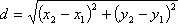### Example Question #1 : Distance Formula

What is the distance between the two points, (1,1) and (7,9)?

7.5

51/2

5

10

3

10

Explanation:

distance2 = (x2 – x1)2 + (y2 – y1)2

Looking at the two order pairs given, x1 = 1, y1 = 1, x2 = 7, y2 = 9.

distance2 = (7 – 1)2 + (9 – 1)= 62 + 82 = 100

distance = 10

### Example Question #4 : Distance Formula

What is the distance between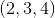and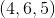?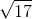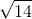Explanation:

distance2 = (x2 – x1)2 + (y2 – y1)2 + (z2 – z1)2

= (4 – 2)2 + (6 – 3)2 + (5 – 4)2

= 22 + 32 + 12

= 14

distance = √14

### Example Question #1 : How To Find The Length Of A Line With Distance Formula

A man travels north 40 meters, while at the same time his wife travels south 20 meters from his initial starting place. He then travels west 100 meters, and his wife travels east 60 meters, followed by him backtracking east 30 meters while his wife stays in the same spot.

Find the approximate value for half the distance between them.

72

220

110

144

56

72

Explanation:

The man travels 40 meters north, 100 meters west, and 30 meters east. After he backtracks, he now has a cumulative distance west of 70 meters and he is 40 meters north. His wife has travelled east 60 meters and south 20 meters. Their positions can be modelled by the following points: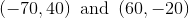We can use the distance fomula to find the distance between the two points.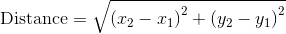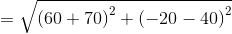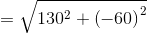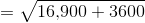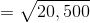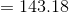Half of this distance would be 71.59, approximately 72 meters.

Tired of practice problems?

Try live online GRE prep today.